• +91 9971497814
• info@interviewmaterial.com

# RD Chapter 16- Surface Areas and Volumes Ex-16.3 Interview Questions Answers

### Related Subjects

Question 1 : A bucket has top and bottom diameters of 40 cm and 20 cm respectively. Find the volume of the bucket if its depth is 12 cm. Also, find the cost of tin sheet used for making the bucket at the rate of Rs 1.20 per dm2.

Given,

Diameter to top ofbucket = 40 cm

So, the radius (r1)= 40/2 = 20 cm

Diameter of bottompart of the bucket = 20 cm

So, the radius (r2)= 30/2 = 10cm

Depth of the bucket(h) = 12 cm

Volume of the bucket =1/3 π(r22 + r12 +rr)h

= π/3(202 +102 + 20 × 10)12

= 8800 cm3

Now,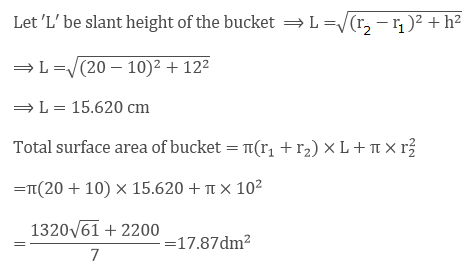Given that the cost oftin sheet used for making bucket per dm2 = Rs 1.20

So, the total costfor 17.87dm2 = 1.20 × 17.87 = Rs 21.40

Therefore, the cost oftin sheet used for making the bucket is Rs 21.40

Question 2 : A frustum of a right circular cone has a diameter of base 20 cm, of top 12 cm and height 3 cm. Find the area of its whole surface and volume.

Given,

Base diameter of cone (d1)= 20 cm

So the radius (r1) = 20/2cm = 10 cm

Top diameter ofCone (d2) = 12 cm

So, the radius (r2) = 12/2cm = 6 cm

Height of the cone (h)= 3 cm

Volume of the frustumof a right circular cone = 1/3 π(r22 + r12 +rr)h

= π/3(102 +6+ 10 × 6)3

= 616 cm3

Let ‘L’ be the slantheight of cone, then we know that

L = √(r1 –r21)2 + h2

L = √(10 – 6)2 +32

L = √(16 + 9)

L = 5cm

So, the slant heightof cone (L) = 5 cm

Thus,

Total surface area ofthe frustum = π(r1 + r2) x L + π r12 +π r22

= π(10 + 6) × 5 +π × 102 + π × 62

= π(80 + 100 +36)

= π(216)

= 678.85 cm2

Question 3 : The slant height of the frustum of a cone is 4 cm and the perimeters of its circular ends are 18 cm and 6 cm. Find the curved surface of the frustum.

Given,

Slant height offrustum of cone (l) = 4 cm

Let ratio of the topand bottom circles be r1 and r2

And given perimetersof its circular ends as 18 cm and 6 cm

2πr1 =18 cm; 2πr2 = 6 cm

πr1=9 cm and πr2 = 3 cm

We know that,

Curved surface area offrustum of a cone = π(r1 + r2)l

= π(r+r2)l

= (πr1+πr2)l =(9 + 3) × 4 = (12) × 4 = 48 cm2

Therefore, the curvedsurface area of the frustum = 48 cm2

Question 4 : The perimeters of the ends of a frustum of a right circular cone are 44 cm and 33 cm. If the height of the frustum be 16 cm, find its volume, the slant surface and the total surface.

Given,

Perimeter of the upperend = 44 cm

2 π r1 =44

2(22/7) r1 =44

r= 7cm

Perimeter of the lowerend = 33 cm

2 π r2 =33

2(22/7) r2 =33

r=21/4 cm

Now,

Let the slant heightof the frustum of a right circular cone be L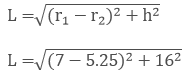L = 16.1 cm

So, the curved surfacearea of the frustum cone = π(r1 + r2)l

= π(7 + 5.25)16.1

Curved surface area ofthe frustum cone = 619.65 cm3

Next,

The volume of thefrustum cone = 1/3 π(r22 + r12 +rr)h

= 1/3 π(72 +5.252 + (7) (5.25) ) x 16

= 1898.56 cm3

Thus, volume of thecone = 1898.56 cm3

Finally, the totalsurface area of the frustum cone

= π(r1 +r2) x L + π r12 + π r22

= π(7 + 5.25) × 16.1 +π7+ π5.252

= π(7 + 5.25) ×16.1 + π(72 + 5.252) = 860.27 cm2

Therefore, the totalsurface area of the frustum cone is 860.27 cm2

Question 5 : If the radii of the circular ends of a conical bucket which is 45 cm high be 28 cm and 7 cm, find the capacity of the bucket

Given,

Height of the conicalbucket = 45 cm

Radii of the 2circular ends of the conical bucket are 28 cm and 7 cm

So, r1 =28 cm r2 = 7 cm

Volume of the conicalbucket = 1/3 π(r12 + r22 +rr)h

= 1/3 π(282 +72 + 28 × 7)45 = 15435π

Therefore, the volume/capacity of the bucket is 48510 cm3.

Question 6 : The height of a cone is 20 cm. A small cone is cut off from the top by a plane parallel to the base. If its volume be 1/125 of the volume of the original cone, determine at what height above the base the section is made.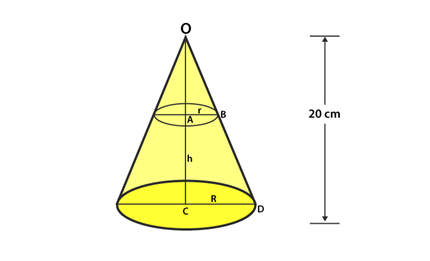Let the radius of thesmall cone be r cm

Radius if the big cone= R cm

Given, height of thebig cone = 20 cm

Let the height ofsection made = h cm

Then, the height ofsmall cone will be = (20 – h) cm

Now,

In OAB and OCD

AOB = COD [common]

OAB = OCD [each 90o]

Then, OAB ~ OCD [by AA similarity]

So, by C.P.S.T we have

OA/ OC = AB/ CD

(20 – h)/ 20 = r/ R ……(i)

Also given,

Volume of small cone =1/125 x volume of big cone

1/3 π r2(20– h) = 1/125 x 1/3 πR2 x 20

r2/ R2 =1/125 x 20/ (20 – h) [From (i)]

(20 – h)2/202 = 1/125 x 20/20 – h

(20 – h)3 =203/ 125

20 – h = 20/5

20 – h = 4

h = 20 – 4 = 16 cm

Therefore, it’s foundthat the section was made at a height of 16 cm above the base.

Question 7 : If the radii of the circular ends of a bucket 24 cm high are 5 and 15 cm respectively, find the surface area of the bucket.

Given,

Height of the bucket(h) = 24 cm

Radius of the circularends of the bucket 5 cm and 15 cm

So, r1 =5 cm; r2 = 15 cm

Let ‘L’ be the slantheight of the bucket

Then, we know that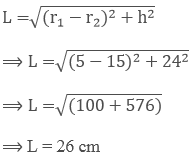Now,

Curved surface area ofthe bucket

= π(r1 +r2)l + πr12

= π(5 + 15)26 +π52 = π(520 + 25) = 545π cm2

Therefore, the curvedsurface area of the bucket = 545π cm2

Question 8 : The radii of circular bases of a frustum of a right circular cone are 12 cm and 3 cm and the height is 12 cm. Find the total surface area and volume of frustum.

Given the height offrustum cone = 12 cm

Radii of a frustumcone are 12 cm and 3 cm

So, r1 =12 cm; r2 = 3 cm

Let slant height ofthe frustum cone be ‘L’

Then, we know that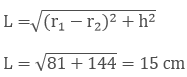L = 15 cm

Now, the total surfacearea of frustum of a cone = π (r1 + r2) x L + π r12 +π r22

= π (12 + 3)15 +π122 + π32

Thus, total surfacearea of the frustum = 378 π cm2

Next,

Volume of frustum cone= 1/3 π(r22 + r12 +rr)h

= 1/3 π(122 +32 + 12 × 3) × 12 = 756π cm3

Therefore, the volumeof the frustum cone = 756 π cm3

Question 9 : A tent consists of a frustum of a cone capped by a cone. If radii of the ends of the frustum be 13 m and 7 m, the height of frustum be 8 m and the slant height of the conical cap be 12 m, find the canvas required for the tent.

Given,

Height of frustum (h)= 8 m

Radii of the frustumcone are 13 cm and 7 cm

So, r1 =13 cm and r2 = 7 cm

Let ‘L’ be slantheight of the frustum cone

Then, we know that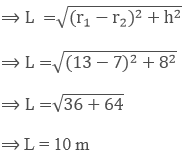Curved surface area ofthe frustum (s1) = π(r1 + r2) × L = π(13 + 7) × 10 = 200 π m2

Then, given slantheight of conical cap = 12 m

Base radius of uppercap cone = 7 m

So, the curved surfacearea of upper cap cone (s2) = πrl = π × 7 ×12 = 264 m2

Thus, the total canvasrequired for tent (S) = s1 + s2

S = 200π + 264 =892.57 m2

Therefore, the canvasrequired for the tent is 892.57 m2.

Question 10 : A milk container of height 16 cm is made of metal sheet in the form of frustum of a cone with radii of its lower and upper ends as 8 cm and 20 cm respectively. Find the cost of milk at the rate of Rs.44 per litre which the container can hold.

Given,

A milk container ofthe form of frustum of a cone

The radius of thelower end (r1) = 8 cm

The radius of theupper end (r2) = 20 cm

Let h be its height, h= 16 cm

Then, the capacity ofthe container = Volume of frustum of the cone

= 1/3 π(r22 +r12 + rr)h

= 1/3 π(202 +82 + (20) (8) ) x 16

= 10459.42 cm3

= 10.46 litres

Now, given that costof 1 litre of milk = Rs 44

Then the cost of 10.46litres of milk = Rs (44 x 10.46) = Rs 460.24

Todays Deals### RD Chapter 16- Surface Areas and Volumes Ex-16.3 Contributorskrishan

Name:
Email:

# Latest News# 9000 interview questions in different categories